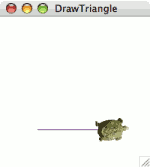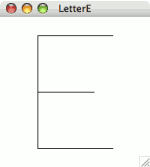# Chapter 3. More on objects

Objects and their classes are central to using Java, which is why Java is called an object-oriented programming language. Before we continue to other concepts, we need to spend a bit more time on how to use objects.

There are many classes that you can use with Java, more than anyone could possibly remember. To use them, you need documentation that lists the methods of each class. To use Java effectively, therefore, you need to understand how to read the documentation.

As an example, let's look at the documentation for the `Turtle` methods that we've seen so far. Recall that our programs have used a constructor for the `Turtle` class as well as two instance methods, `forward` and `hide`. Here's the documentation for them.

`Turtle(double x, double y)`

(Constructor) Constructs a turtle who is initially `x` pixels from the left side of the drawing area and `y` pixels from the top. The turtle will initially face east.

`void forward(double dist)`

Moves this turtle forward `dist` pixels in its current direction, tracing a line along the path.

`void hide()`

Removes this turtle from its drawing area. The lines it has traced remain.

For now, ignore the word `void` written before `forward` and `hide` above; we'll get to it in the next chapter.

Recall that a constructor is used for creating a new object of that type, using the keyword `new` followed by the class name. A constructor is always named the same as the class; thus, in the `Turtle` class documentation, the constructor is listed as `Turtle(``)`.

The constructor and the `forward` method both take parameters, which are described in the above documentation in a rather cryptic way. In listing the `forward` method, it says the following.

`void forward(double dist)`

Moves this turtle forward `dist` pixels in its current direction, tracing a line along the path.

Inside the parentheses you see `double dist`. This syntax is akin to the variable declaration syntax as in line 5 of `DrawLine` (`Turtle yertle;`): You have a type, followed by a name. In this case, we have `double dist` — the parameter, named `dist`, is of the `double` type.

The name `dist` is really irrelevant — it's only there so that the English description of the method can refer to it. (This is useful for methods taking multiple parameters, as with the constructor.) The type `double` is important, though. The `double` type is Java's type for numbers. Thus, this documentation says that the `forward` method requires a number as a parameter.Java's designers chose `double` as the name for the numeric type for historical reasons. Its predecessor, C, used `double` to indicate a number that is stored in twice as much memory as regular numbers. The added memory allows the computer to remember the number more precisely (with 15 digits of precision, instead of merely 6) and to remember larger numbers. Over the years, as memory became cheaper, programmers began using `double` almost exclusively, since there was little reason to risk erroneous results in order to skimp on memory.

You can see a similar thing with the constructor: It takes two parameters, both numbers. The English description tells us that these will be the turtle's initial coordinates. As the documentation for the constructor states, when a turtle is newly created, it is facing east.

## 3.2. Using class documentation

contains documentation for all of the classes and methods in the `turtles` package. We'll introduce each item in the text as it becomes needed, but the appendix is a handy reference.

Right now, let's look at three more methods in the `Turtle` class that you would also be able to find in .

`void left(double angle)`

Turns this turtle `angle` degrees counterclockwise.

`void right(double angle)`

Turns this turtle `angle` degrees clockwise.

`void setPenColor(Color value)`

Changes the pen color to `value`. The turtle will use this color for drawing future lines.

The first two indicate that turtles can be told to turn `left` or `right`; both of these methods require a number as a parameter. The last method, `setPenColor` requires a `Color` parameter. We haven't seen this type before, but we can learn all we need to know about it by reading its documentation.

`Color(int r, int g, int b)`

(Constructor) Constructs an object representing a color, using proportions of red, green, and blue as specified in `r`, `g`, and `b` respectively. The three parameters must all be integers between 0 and 255. For white, use 255 for all parameters; for black, use 0 across the board.

Now let's look at a new program combining these extra methods. Figure 3.1 contains a program to draw a mauve triangle.

Figure 3.1: The `DrawTriangle` program.

```  1  import java.awt.*;   2  import turtles.*;   3     4  public class DrawTriangle extends TurtleProgram {   5      public void run() {   6          Color mauve;   7          mauve = new Color(102, 51, 128);   8     9          Turtle ramses;  10          ramses = new Turtle(50, 150);  11          ramses.setPenColor(mauve);  12          ramses.forward(100);  13          ramses.left(120);  14          ramses.forward(100);  15          ramses.left(120);  16          ramses.forward(100);  17          ramses.hide();  18      }  19  } ```

Figure 3.2: Running `DrawTriangle`.

(a) (b) (c)(d) (e)First, notice the addition of line 1. We need this new `import` line because this program uses the `Color` class, and the `Color` class is part of the `java.awt` library (called a package in Java-speak).

Let's trace what happens when the computer runs this program.

1. Line 6: Declares a variable `mauve` that can potentially refer to a `Color` object.

2. Line 7: Creates a new `Color` object that has about 40% of the maximum red value (of 255), 20% of the maximum green value, and 50% of the maximum blue value. This color has relatively little of all three primary hues, so it is fairly dark. But it is primarily a mixture of red and blue, so we can guess it will be a dark purplish color — or, to be more precise, mauve.

3. Line 9: Declares a variable `ramses` that can potentially refer to a `Turtle` object.

4. Line 10: Creates a new `Turtle` object at (50, 150) and assigns `ramses` to be a name for this object. Figure 3.2(a) illustrates how the window will look at this point.

5. Line 11: Tells `ramses` to change its pen color to what is specified in the `Color` object created in line 7.

6. Line 12: Tells `ramses` to execute its `forward` method, with 100 as a parameter. Since the turtle is facing east, this will move it from (50, 150) to (150, 150). The turtle traces a line, which will form the base of the triangle. See Figure 3.2(b).

7. Line 13: Tells `ramses` to execute its `left` instance method, with 120 as a parameter. As the documentation for the `Turtle` class indicates, its `left` method's parameter specifies how many degrees the turtle should turn counterclockwise. So, while the turtle was facing at 3 o'clock before, now it is facing 120° counterclockwise of that, at 11 o'clock.

8. Line 14: Tells `ramses` to go `forward` 100 pixels. This draws the right side of the triangle. See Figure 3.2(c).

9. Line 15: Tells `ramses` to turn 120 degrees counterclockwise. The turtle is now facing at 7 o'clock.

10. Line 16: Tells `ramses` to move `forward` 100 pixels. This draws the left side of the triangle. See Figure 3.2(d).

11. Line 17: Tells `ramses` to `hide`. The path the turtle traced, however, remains, leaving us with a mauve triangle. See Figure 3.2(e).

## 3.3. Multiple names for the same object

Figure 3.3 contains a program that's a little confusing. This program is a bit contrived, but it illustrates several points about how objects work that are important to being able to use Java proficiently. Among other things, it illustrates how Java works when a program assigns multiple variables to refer to the same object.

Before we examine this program step by step, try tracing through yourself to figure out what you expect it to do, and sketch the result on a sheet of paper.

Figure 3.3: The `TurtleRace` program.

```  1  import turtles.*;   2     3  public class TurtleRace extends TurtleProgram {   4      public void run() {   5          Turtle upper;   6          Turtle cur;   7          cur = new Turtle(10, 60);   8          upper = cur;   9          cur.forward(90);  10          cur = new Turtle(10, 140);  11          cur.forward(90);  12          cur = upper;  13          cur.forward(90);  14      }  15  } ```

Here's how the computer will execute the program.

1. Lines 5–6: Creates two `Turtle` variables, named `upper` and `cur`.

2. Line 7: Creates a turtle at (10, 60), to which `cur` refers. We'll also call this Turtle A for the purposes of our discussion.

3. Line 8: Assigns `upper` to refer to the same turtle as `cur`; since `cur` currently refers to Turtle A, so will `upper`.

4. Line 9: Tells `cur` (which is Turtle A) to move forward 90 pixels. Now Turtle A is at (100, 60).

5. Line 10: Reassigns `cur` to be a new `Turtle` object located at (10, 140), which we'll name Turtle B. Since line 14 does not change `upper`, and `upper` was referring to Turtle A from before, `upper` still refers to Turtle A.

6. Line 11: Tells `cur` (which refers to Turtle B) to move forward 90 pixels. Now Turtle B is at (100, 140).

7. Line 12: Assigns `cur` to refer to the same turtle as `upper`; since `upper` currently refers to Turtle A, so will `cur`.

8. Line 13: Tells `cur` (which refers to Turtle A) to move forward 90 pixels. Now Turtle A is at (190, 60).

Thus, at the end of the program, there are two turtles in the window. One (Turtle A) has moved from (10, 60) to (190, 60). And the other (Turtle B) has moved from (10, 140) to (100, 140). Figure 3.4 illustrates the final result.

Figure 3.4: Running `TurtleRace`.Exercise 3.1

Without running the program on a computer, draw a picture of what the `TurtleMystery` program of Figure 3.5 would draw on the screen.

Figure 3.5: The `TurtleMystery` program.

```  1  import turtles.*;   2     3  public class TurtleMystery extends TurtleProgram {   4      public void run() {   5          Turtle squishy;   6          squishy = new Turtle(50, 150);   7          squishy.right(90);   8          squishy.forward(30);   9          squishy.left(90);  10          squishy.forward(40);  11          squishy.left(90);  12          squishy.forward(30);  13          squishy.hide();  14    15          squishy = new Turtle(70, 30);  16          squishy.right(45);  17          squishy.forward(30);  18          squishy.hide();  19    20          squishy = new Turtle(70, 30);  21          squishy.right(135);  22          squishy.forward(30);  23          squishy.hide();  24      }  25  } ```

Exercise 3.2

Insert code into the `TurtleRace` program of Figure 3.3, so that both turtles hide themselves after Turtle A makes its last move (line 13). You may only add to the program; do not delete or modify any of the code already in the program. To accomplish this, you will need to add a new variable to remember Turtle B, since both `upper` and `cur` refer to Turtle A after line 12.

Exercise 3.3

Write a program to draw the capital letter E, as in Figure 3.6. In the screen shot, the letter is 120 pixels wide and 140 pixels tall.

Figure 3.6: Drawing the capital letter E.